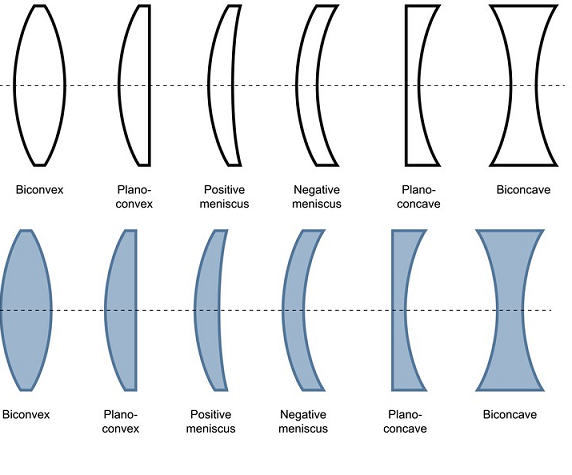## Grade 7  |   Science  |   Lens, Light, Olympiad, CBSE, ICSE, SOF, ITO

`Lens`

A lens is a curved piece of glass or plastic designed to refract light in a specific way. Lenses are used in glasses and contacts to help correct vision.

• Convex - A convex lens is one where the center of the lens is thicker than the edges.
• Concave - A concave lens is one where the center of the lens is thinner than the edges. One way to remember the difference between the two lenses is to think of "caving in" with the concave lens.
•Basic terms related to lens

Focal Point

The focal point of a lens is generally noted by the capital letter "F." This is the point in space where the light rays will converge to after passing through a converging lens. A diverging lens will have a negative focal point where the rays originate from before diverging through the lens.

Focal Length

The focal length is the distance from the center of the lens to the focal point.

Principal Axis

The principal axis is a horizontal imaginary line drawn through the center of the lens. In a perfect lens the focal point will reside on the principal axis at a distance of the focal length from the center of the lens.